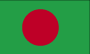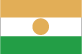# Lab Exercise for Assignment 1: Input, Output, Variables.

## COMP112 2020 Tri 1: Lab Exercise 1

#### Goals

The goal of this assignment is for you to be able to construct programs in Java that
• use methods to get simple user input
• use variables for storing values, and expressions for computing values.
• use methods for output, both text and graphics.

#### Overview

The exercises for this lab involve writing programs very similar to the ones shown in lectures.

#### Preparation

Download the zip file for lab exercise 1 and extract it to the `COMP112-2020T1-Lab1` folder in your home folder. It should contain templates for the exercises program. Read through the exercises below to see what you need to do. In order to see what your programs should do, you can run the demo programs (on the ECS lab computers - they should be in the COMP112 folder on your desktop).

#### Summary

There are two parts to the exercises:
• Calculators exercise: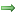Write programs to convert miles to kilometers, grams to ounces, Kelvin to Fahrenheit, and calculate the area of a triangle, the area of the surface of a sphere, and the cost of peppers.
• Flags exercise:Write programs to draw several simple flags.

#### Structure of the Exercises

Each part is a series of very small programs to check that you understand the key principles. You should write these programs in the lab session while the tutors are there to help. It is just fine to get the tutors or other students to help you when working on an exercise program - that's how you will learn the key ideas in the course. You do not need to do all the exercises - you can stop a series once you have completed a program in the series without help.

### Exercise Series 1: Calculators.

For the first series of exercises, you must complete methods that perform a simple calculation. Each method should ask the user for one or two numbers, and print out the answer of the computation. The `CalculatorExercise` demo shows what they should do.

• Video demonstrating what your CalculatorExercise program should do

The file `CalculatorExercise.java` contains one method called `milesToKilometers` that currently does nothing. You should complete the method so that it converts miles to kilometers:
• Ask the user for the number of miles
• Compute the number of kilometers (there are 1.609 kilometers in a mile)
• Print out the number of kilometers eg = 45.783 kilometers

Write similar methods ( `triangleArea` , `gramsToOunces` , etc) to perform the following calculations. You may stop once you have written one of the methods by yourself.
• Ask the user for the base and the height of a triangle, and print out the area (half the product of the base and the height).
• Ask the user for grams and print out the number of ounces.
• Ask the user for the radius of a sphere and print out the surface area (4 * radius2 * π) and the volume (4/3 * radius3 * π)
• Ask the user for the temperature in degrees Kelvin and print out the temperature in Fahrenheit (fahrenheit is kelvin * 9/5 - 459.67)
• Ask the user for the number of pizzas, and the cost per pizza and print out the total cost (in dollars).

### Exercise Series 2: Flags.

For the second series of exercises, you must complete methods that draw various simple national flags. The `FlagExercise` demo shows what they should do.

• Video showing what your FlagExercise programme should do.

The file `FlagExercise.java` contains two constants, `LEFT` and `TOP`, which specify the position of the flags.

The first method is called `drawJapanFlag`. It should clear the graphics pane and ask the user for the width of the flag, then calculate the height of the flag, the circle diameter, and the left and top of the circle. Finally, it should draw the flag. It should use the constants `LEFT` and `TOP` to work out where the flag is placed.

 Japan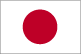instructions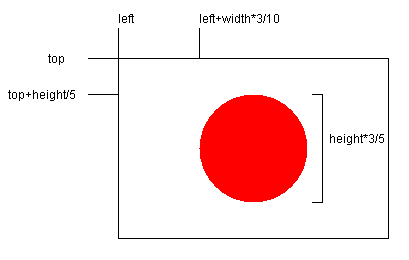Test your method with at least two different widths.

Write `drawIndonesiaFlag` to draw the flag of Indonesia.
 Indonesia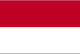Write similar methods (`drawAustriaFlag` , etc) to draw the flags of the following countries. You may stop once you have written one of the flag methods by yourself.

 Austria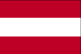instructions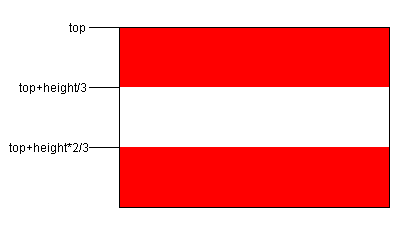Bangladesh Niger